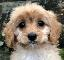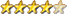Simple Maze using Truchet Tiles

Author Message
TassyJimGuruJoined: 07/08/2011
Location: Australia
Posts: 5423
 Posted: 02:14am 03 Apr 2021

Very nice.
To go one step further, I added mouse control

Click anywhere to show the connected paths

' Maze formed from Truchet Tiles
' This is basically the same algorithm as the famous
' Commodore Pet '10Print' one-line maze.
' Rev 1.0.0 William M Leue 4/1/2021
OPTION DEFAULT INTEGER
OPTION BASE 1
DIM INTEGER oldX, newX, oldY, newY
CONST TSIZE = 30
CORNERS = 0
mouse_port = MM.INFO(OPTION MOUSE)
IF mouse_port <> -1 THEN ' we have a mouse
CONTROLLER MOUSE OPEN mouse_port
GUI CURSOR ON 0,MOUSE(x,mouse_port), MOUSE(y,mouse_port)
ENDIF
DO
CLS
ncols = MM.HRES\TSIZE
nrows = MM.VRES\TSIZE
FOR row = 1 TO nrows+1
y = (row-1)*TSIZE
FOR col = 1 TO ncols+1
x = (col-1)*TSIZE
DrawTruchetTile x, y, RND()
NEXT col
NEXT row
BOX 0,0,MM.HRES,MM.VRES

DO : k\$ = INKEY\$
GUI CURSOR MOUSE(X, mouse_port), MOUSE(Y, mouse_port)
IF MOUSE(L,mouse_port) THEN mouseclick
LOOP UNTIL k\$ <> ""
IF k\$ = "1" THEN CORNERS = 1
IF k\$ = "0" THEN CORNERS = 0
LOOP UNTIL k\$ = CHR\$(27)

IF mouse_port <> -1 THEN ' we have a mouse
CONTROLLER MOUSE CLOSE mouse_port
GUI CURSOR OFF
ENDIF
END

SUB DrawTruchetTile x, y, r AS FLOAT
IF CORNERS THEN
IF r < 0.5 THEN
LINE x + TSIZE/2, y, x + TSIZE, y + TSIZE/2
LINE x, y + TSIZE/2, x + TSIZE/2, y + TSIZE
ELSE
LINE x + TSIZE/2, y, x, y + TSIZE/2
LINE x + TSIZE, y + TSIZE/2, x + TSIZE/2, y + TSIZE
END IF
ELSE
IF r < 0.5 THEN
ARC x, y + TSIZE, TSIZE/2,, 0, 93
ARC x + TSIZE, y, TSIZE/2,, 180, 273
ELSE
ARC x, y, TSIZE/2,, 87, 180
ARC x + TSIZE, y + TSIZE, TSIZE/2,, -93, 0
END IF
END IF
END SUB

SUB mouseClick
newX = INT((MOUSE(X, mouse_port)+tsize/2)/tsize)*tsize
newY = INT((MOUSE(Y, mouse_port)+tsize/2)/tsize)*tsize
GUI CURSOR HIDE
IF oldX <>0 THEN
PIXEL FILL oldX,oldY,RGB(BLUE) ' hide the red dot
PIXEL FILL oldX,oldY,RGB(BLACK)
ENDIF
PIXEL FILL newX,newY,RGB(BLUE)
CIRCLE newX,newY,TSIZE/4,0,1,,RGB(RED)
oldX = newX
oldY = newY
GUI CURSOR SHOW
END SUB

I have assumed that you have a mouse set with OPTION MOUSE n

Jim